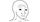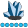somebody
Lapis Lazuli | Level 10

## convert 3 column dataset into matrix

I have the following input:

 Ticker1 Ticker2 Edge ABC EFG 3 ABC NOM 10 ABC XYZ 50 EFG NOM 2 EFG XYZ 0 EFG QQQ 2 XYZ QQQ 6 XYZ NOM 99

Which I would like to convert to an n x n matrix:

 ABC EFG NOM XYZ QQQ ABC 3 10 50 EFG 3 2 0 2 NOM 10 2 99 6 XYZ 50 0 99 QQQ 2 6

How should I do this? the above is just to give an idea. My input dataset is:

``````data WORK.CLASS;
infile datalines dsd truncover;
input ticker1:\$10. ticker2:\$10. edge:32.;
datalines4;
ABC,EFG,3ABC,NOM,10ABC,XYZ,50EFG,NOM,2EFG,XYZ,0EFG,QQQ,2XYZ,QQQ,6XYZ,NOM,99
;;;;``````

I cut this down to 15 obs but my dataset has 500 and the ticker set are the same for ticker1 and ticker2. Thus I would want a n x n matrix.

8 REPLIES 8

## Re: convert 3 column dataset into matrix

To clarify:

1. You say "the ticker symbols are the same for ticker1 and ticker2." Does that mean that you have a long data set that has (n x n) rows and you want to convert it to an (n x n) matrix? Or do you only have the upper triangular portion of the matrix, as shown in the little 3x3 example, which has  3 observation?

2. In the second data set, do the cell counts from the Position variable? Is the Edge variable used at all?

In your example, Ticker1 and Ticker2 do not have the same symbols. Please provide sample data that fits the problem you want to solve (or adjust your description of the problem)somebody
Lapis Lazuli | Level 10

## Re: convert 3 column dataset into matrix

1. What I meant was ticker1 and ticker2 contains tickers from a set of tickers. If you are familiar with networks, this dataset is an edge list for a network and each observation specifies the weighted connection between 2 nodes (vertices).

2. Sorry there should not be a position variable. I have removed it in the question. Regarding the second question, the Edge variable is used for the values of the matrix.  So its similar to correlation between 2 tickers in the correlation matrix.

I have updated the question.

## Re: convert 3 column dataset into matrix

Another question:

Look at the output of the following call to PROC FREQ. Is this what you want, except you want it in a symmetric matrix in SAS/IML?

``````proc freq data=class;
tables ticker1*ticker2 / norow nocol nocum nopercent;
weight Position;
run;``````

## Re: convert 3 column dataset into matrix

The way to solve this problem depends on properties of the input data:

1. Are the data sorted by Ticker1 Ticker2 ?

2. Are all joint levels represented? That is, do you have an observation for every possible pair?

If the answers to (1) and (2) are both yes, then I think the following DATA step produces sample data that meets the conditions. The SAS/IML statement reads the data into the upper triangular portion of a matrix and then makes the matrix symmetric. The program uses the ROW and COL function calls, which were introduced in SAS/IML 13.1 (SAS 9.4M1).

``````data Have;
array c \$ ('A','B','C','D','E');
do i = 1 to dim(c);
Var1 = c[i];
do j = i+1 to dim(c);
Var2 = c[j];
Count = floor(10*uniform(1));
output;
end;
end;
keep Var1 Var2 Count;
run;

proc iml;
/* assume Have is sorted by (Var1, Var2) and that
all joint levels are represented (even zero counts) */
use Have;
read all var {Var1 Var2 Count};
close;

u = union(var1, var2);
n = ncol(u);
M = j(n, n, 0);                   /* allocate matrix of zeros */
upperTriIdx = loc(row(M) < col(M)); /* upper triangular indices */
M = M + M`;               /* make symmeetric */
print M[r=u c=u];``````

## Re: convert 3 column dataset into matrix

@Rick_SAS - A minor quibble.  Does not

loc(row(M) > col(M));

generate indices for lower (rather than upper) triangle?

--------------------------
The hash OUTPUT method will overwrite a SAS data set, but not append. That can be costly. Consider voting for Add a HASH object method which would append a hash object to an existing SAS data set

Would enabling PROC SORT to simultaneously output multiple datasets be useful? Then vote for
Allow PROC SORT to output multiple datasets

--------------------------

## Re: convert 3 column dataset into matrix

Thanks, Mark. I have changed the inequality to match the comment.

## Re: convert 3 column dataset into matrixsomebody
Lapis Lazuli | Level 10

## Re: convert 3 column dataset into matrix

Sorry, I edited it.
From The DO Loop